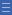## Featured resource

Home > Topdrawer > Mental computation > Activities > Up through ten

# Up through ten

Up through ten

Up-through-ten strategies use decades as landmarks to solve harder calculations. For example, 9 + 7 = ? can be solved by calculating 9 + 1 = 10 then 10 + 6 = 16.

A bead string is an excellent linear representation for developing the up-through-ten strategy.

Clearly mark the decades on the bead string and arrange the beads in sets of five. This supports non-counting-by-one strategies. Knowing the number facts for the counting numbers to 10 is essential. You can watch the Up-Through-Ten video.

Up-through-ten strategies can be practised using the learning object L91 The part-adder: make your own easy sums.

This object uses a continuous model with the ones grouped within each bar.

Highlight the characteristics of problems where the up-through-ten strategy is or is not needed.

For example, 8 + 6 = ? is suitable as only two more are required to make up to the next decade. However, 6 + 5 is not suitable and is more appropriately solved using other strategies like near doubles.

These ideas are further explored in Number Facts: Addition and Subtraction and Place Value.

Yes

Yes

Name Class SectionYear 2: Solve simple addition and subtraction problems using a range of efficient mental and written strategies Infobox 3Year 3: Recall addition facts for single-digit numbers and related subtraction facts to develop increasingly efficient mental strategies for computa... Infobox 3Source Infobox 3